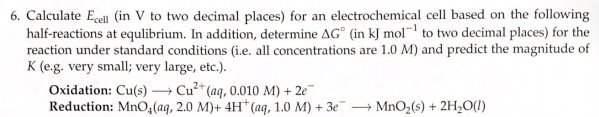# 6. Calculate Ecell (in V to two decimal places) for an electrochemical cell based on the following half-reactions at equilibrium. In addition, determine ΔG° (in kJ mol^-1 to two decimal places) for the reaction under standard conditions (i.e. all concentrations are 1.0 M) and predict the magnitude of K (e.g. very small; very large, etc.). Oxidation: Cu(s) → Cu^2+(aq, 0.010 M) + 2e^- reduction: MnO4(aq, 2.0 M)+ 4H^+(aq, 1.0 M) + 3e^- → MnO2(s) + 2H2O(l)